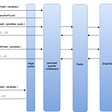# DS with JS — Linked Lists — II

## Data Structures with JavaScript — Chapter Two — Doubly Linked Lists

A doubly linked list is a linked data structure that consists of a set of sequentially linked records called nodes. Each node contains two fields, called links, that are references to the previous and to the next node in the sequence of nodes.

## Before Proceeding further…

This post is continued from DS with JS — Linked Lists, If you haven’t gone through that yet, make sure you do before proceeding further…

# ⚜️ The list

1. Append
2. AppendAt
3. Remove
4. RemoveAt
5. Reverse
6. Swap
7. IsEmpty & Length
8. Traverse
9. Display
10. Search

# Lets’ begin!!

All the code snippets you will see below are just Pseudo code. If you want to see working code — click here

## 🔅 Append

`append( item ) {  let node = new Node( item );  if(!this.head) {    this.head = node;    this.tail = node;  } else {    node.prev = this.tail;    this.tail.next = node;    this.tail = node  }}`

## 🔅 AppendAt

`appendAt( pos, item ) {   let current = this.head;   let counter = 1;   let node = new Node( item );   if( pos == 0 ) {     this.head.prev = node     node.next = this.head     this.head = node   } else {     while(current) {      current = current.next;      if( counter == pos ) {        node.prev = current.prev        current.prev.next = node        node.next = current        current.prev = node      }      counter++    }  }}`

## 🔅 Remove

`remove( item ) {  let current = this.head;  while( current ) {    if( current.data === item ) {      if( current == this.head && current == this.tail ) {        this.head = null;        this.tail = null;      } else if ( current == this.head ) {        this.head = this.head.next        this.head.prev = null      } else if ( current == this.tail ) {        this.tail = this.tail.prev;        this.tail.next = null;      } else {        current.prev.next = current.next;        current.next.prev = current.prev;      }   }   current = current.next  }}`

## 🔅 RemoveAt

`removeAt( pos ) {  let current = this.head;  let counter = 1;  if( pos == 0 ) {   this.head = this.head.next;   this.head.prev = null;  } else {   while( current ) {    current = current.next    if ( current == this.tail ) {     this.tail = this.tail.prev;     this.tail.next = null;    } else if( counter == pos ) {     current.prev.next = current.next;     current.next.prev = current.prev;     break;    }    counter++;   }  }}`

## 🔅 Reverse

`reverse(){  let current = this.head;  let prev = null;  while( current ){   let next = current.next   current.next = prev   current.prev = next   prev = current   current = next  }  this.tail = this.head  this.head = prev}`

## 🔅 Swap

`swap( nodeOne, nodeTwo ) {   let current = this.head;   let counter = 0;   let firstNode;   while( current !== null ) {     if( counter == nodeOne ){       firstNode = current;     } else if( counter == nodeTwo ) {       let temp = current.data;       current.data = firstNode.data;       firstNode.data = temp;     }     current = current.next;     counter++;   }   return true}`

## 🔅 IsEmpty & Length

`length() {  let current = this.head;  let counter = 0;  while( current !== null ) {   counter++   current = current.next  }  return counter;}isEmpty() {  return this.length() < 1}`

## 🔅 Traverse

`traverse( fn ) {   let current = this.head;   while( current !== null ) {    fn(current)    current = current.next;   }   return true;}`

## 🔅 TraverseReverse

`traverseReverse( fn ) {  let current = this.tail;  while( current !== null ) {   fn(current)   current = current.prev;  }  return true;}`

## 🔅 Search

`search( item ) {  let current = this.head;  let counter = 0;  while( current ) {    if( current.data == item ) {     return counter    }    current = current.next    counter++  }  return false;}`

# One more important thing..

This post is the second instalment to its series “DS with JS”. There will be more in this series. Next will be on next Thursday Morning. Stay tuned!

# That’s It

## Happy Coding !!

🎧 No music today… I lost something very important today…

If you like this article, please give it some claps 👏 and share it! If you do not like this post or have any type of questions regarding anything that i mentioned in this post. Feel free to ask me. Just post an issue in my “Ask Me Anything” by clicking here.

--

--

--

## More from _devblogs

Stories for Full-Stack Web developers which help them in pursuing their goals as a developer, mastering the modern web technologies and *hacking the web.

## Promises in JavaScript## useRef vs. useState in React## GraphQL Dynamic Persisted Queries## How we optimised our indiagold react app to improve our PageSpeed performance score## Increase code quality with Github Actions## Demystifying The Big O(bsession) Part II## The One JavaScript Library I Almost Always Use## Gaurav Mehla

Software engineer & Web hacker. Spent 30+% of life on playing with JS

## Server-Sent Event (SSE) Chat Application using Spring Boot and React Js## An Extremely Basic Introduction into Websockets## Graph Search in JS: Depth First Search — 3